# Metrics comparison

The results are reported for the testing portion of the dataset: randomly selected 80% of the conditions. The remaining 20% was used to calibrate FovVideoVDP parameters. The testing set was used to fit JOD regression (JOD regression is not a part of training). The scatter plots and detailed reports (if present) show the entire dataset.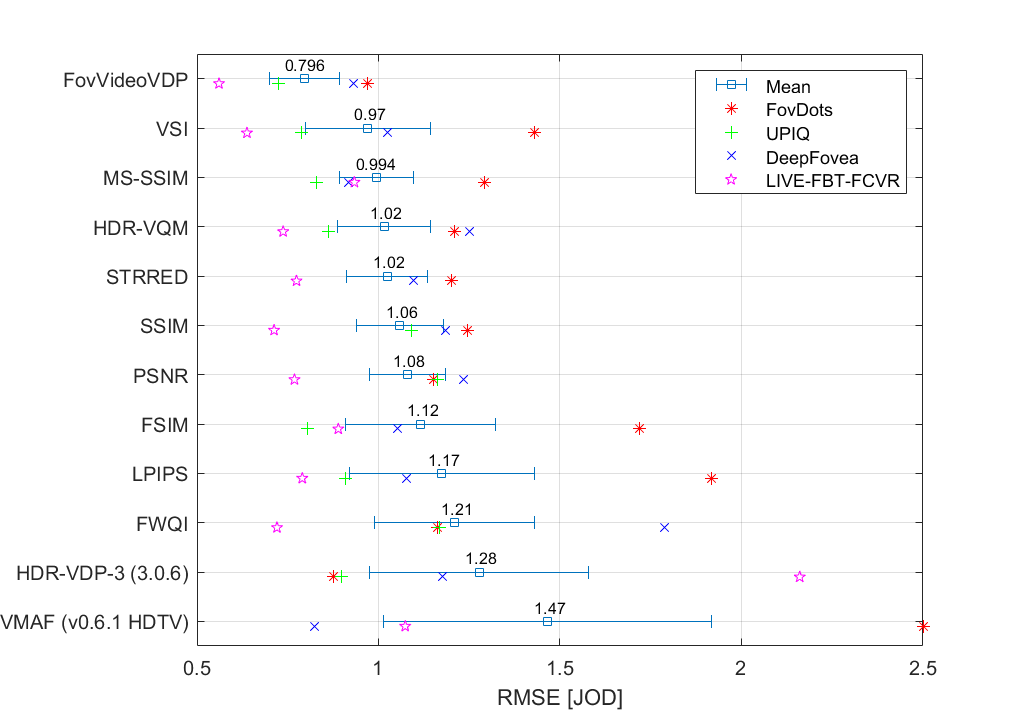Metric/Variant RMSE PLCC SROCC FovDots UPIQ DeepFovea LIVE-FBT-FCVR Average(1) FovDots UPIQ DeepFovea LIVE-FBT-FCVR Average(2) FovDots UPIQ DeepFovea LIVE-FBT-FCVR Average(3) FovVideoVDP → 0.9703 0.7232 0.9308 0.5606 0.7962 0.722 0.8745 0.8136 0.7999 0.8697 0.769 0.8493 0.8423 0.7913 0.8492 VSI → 1.429 0.7884 1.025 0.6377 0.9702 0.7481 0.851 0.828 0.8489 0.8395 0.7702 0.8289 0.7966 0.8525 0.8257 MS-SSIM → 1.292 0.8304 0.9185 0.9336 0.9937 0.7563 0.8389 0.8412 0.8011 0.8249 0.7835 0.8334 0.8378 0.8085 0.8239 HDR-VQM → 1.21 0.8626 1.251 0.7374 1.015 0.6954 0.8154 0.761 0.6684 0.8045 0.7402 0.7675 0.7412 0.6749 0.7613 STRRED → 1.201 NaN 1.097 0.7741 1.024 0.8104 NaN 0.8214 0.6532 0.5948 0.8266 NaN 0.8248 0.656 0.6126 SSIM → 1.247 1.092 1.184 0.7123 1.059 0.6073 0.6842 0.7945 0.6579 0.6798 0.6112 0.7134 0.779 0.6663 0.7129 PSNR → 1.152 1.163 1.235 0.7684 1.079 0.5801 0.6255 0.723 0.5917 0.6229 0.5577 0.6702 0.7152 0.5583 0.6662 FSIM → 1.719 0.8032 1.052 0.8892 1.116 0.7475 0.8595 0.7492 0.7615 0.8385 0.8632 0.8032 0.7429 0.7742 0.7937 LPIPS → 1.919 0.9093 1.078 0.7899 1.174 0.6385 0.7937 0.7927 0.8451 0.7716 0.6671 0.7722 0.7834 0.8442 0.7554 FWQI → 1.161 1.169 1.789 0.7203 1.21 0.6324 0.6216 0.02874 0.7606 0.6085 0.6621 0.6291 0.2125 0.7448 0.6191 HDR-VDP-3 (3.0.6) → 0.8748 0.8969 1.176 2.16 1.277 0.8553 0.8129 0.692 0.7161 0.7432 0.8615 0.8084 0.6881 0.747 0.7799 VMAF (v0.6.1 HDTV) → 2.5 NaN 0.8232 1.074 1.466 0.657 NaN 0.8648 0.7919 0.7841 0.7381 NaN 0.861 0.79 0.7784

(1)Average RMSE is computed as an average of RMSEs of individual datasets so that each dataset has the same influence on the average RMSE regardless of the number of conditions it contains.

(2-3)Average correlation coeffcients are computed for the consolidated dataset consisting of all four individual datasets. It means that the average correlation coefficients are dominated by the performance on UPIQ, which contains over 4000 conditions. For that reason, RMSE is more indicative of metric performance.

## FovVideoVDP

RMSE = 0.7962 PLCC = 0.8697 SROCC = 0.8492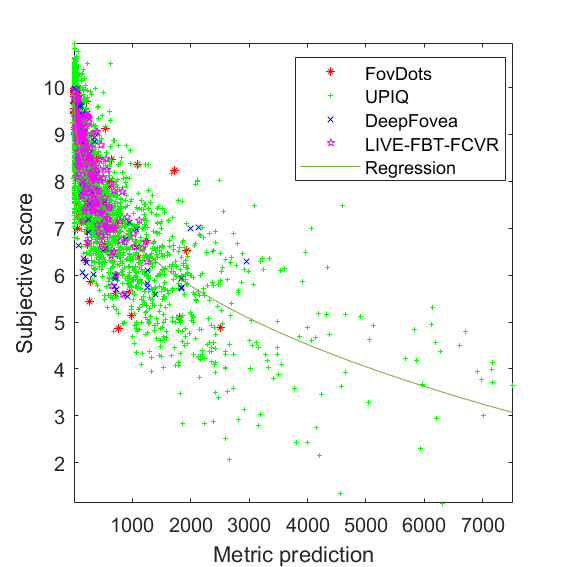JOD regression: Q = 10 -0.249449 * M^0.372455

## VSI

RMSE = 0.9702 PLCC = 0.8395 SROCC = 0.8257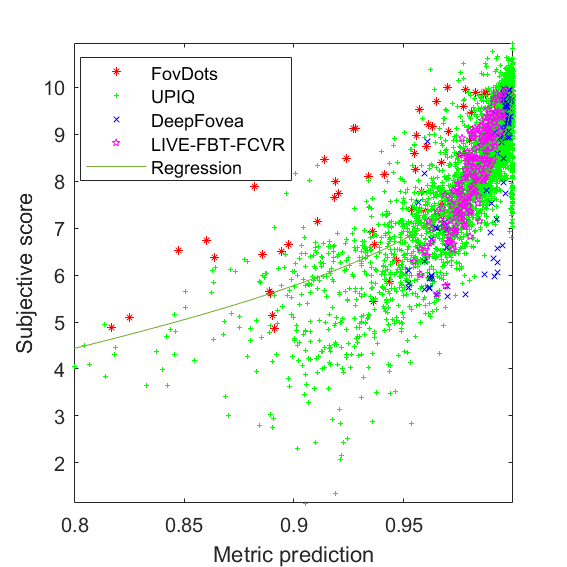JOD regression: Q = -120.401 * z^0.0301661 + 10, z=log2(1.001-M)

## MS-SSIM

RMSE = 0.9937 PLCC = 0.8249 SROCC = 0.8239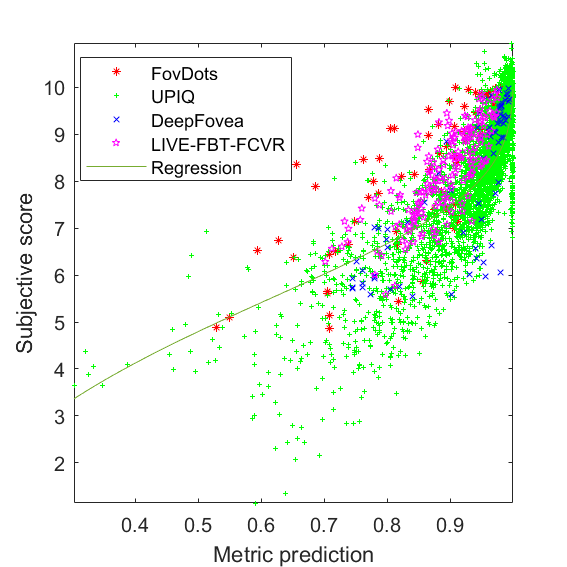JOD regression: Q = -102.849 * z^0.0214474 + 10, z=log2(1.001-M)

## HDR-VQM

RMSE = 1.015 PLCC = 0.8045 SROCC = 0.7613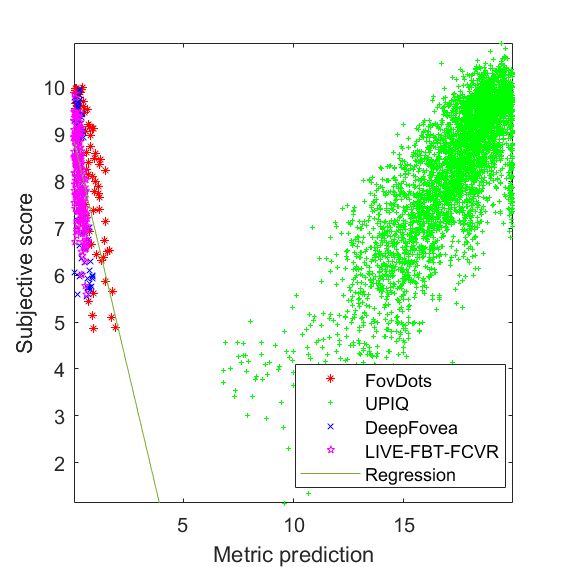JOD regression: Q = -1.98843 * M + 8.91004

## STRRED

RMSE = 1.024 PLCC = 0.5948 SROCC = 0.6126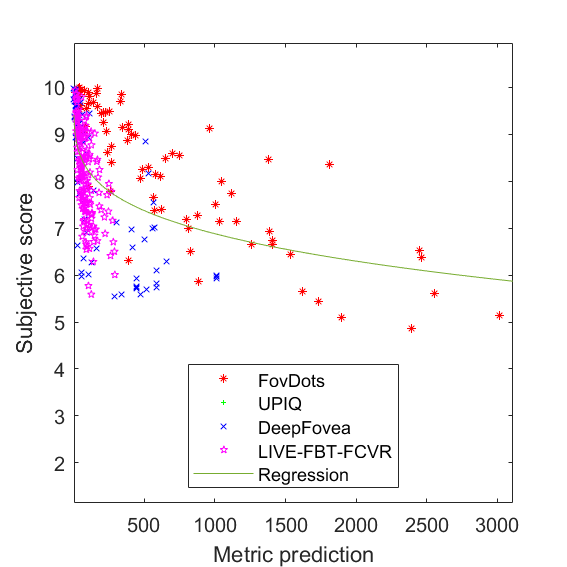JOD regression: Q = 10 -0.564865 * M^0.247371

## SSIM

RMSE = 1.059 PLCC = 0.6798 SROCC = 0.7129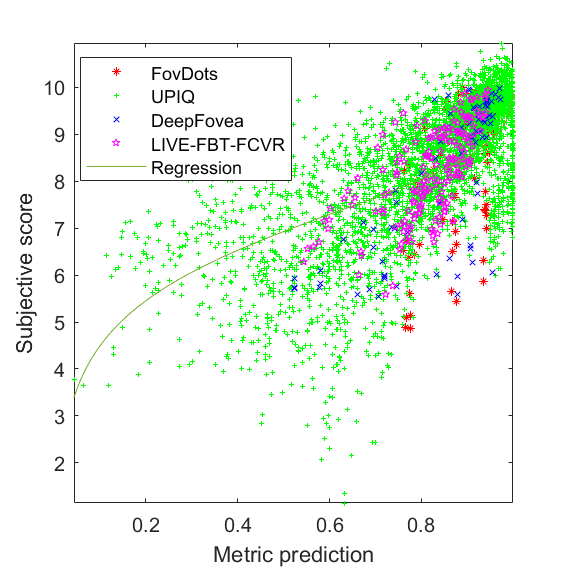JOD regression: Q = -83.2433 * z^0.0157508 + 10, z=log2(1.001-M)

## PSNR

RMSE = 1.079 PLCC = 0.6229 SROCC = 0.6662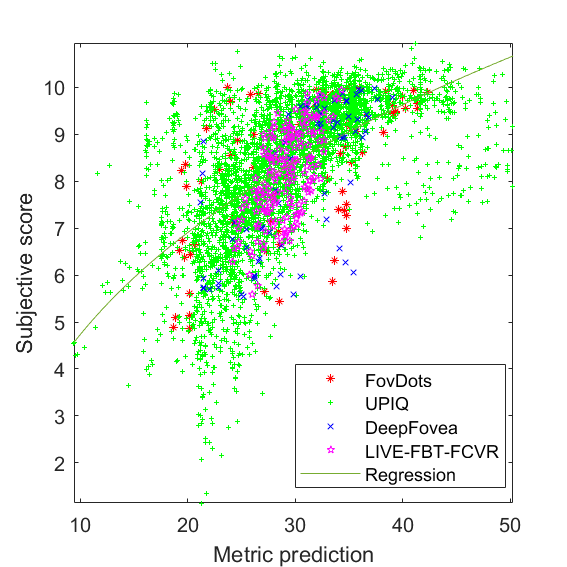metric to JOD mapping params: Q = 4.06072 * M^0.325177 + -3.84643

## FSIM

RMSE = 1.116 PLCC = 0.8385 SROCC = 0.7937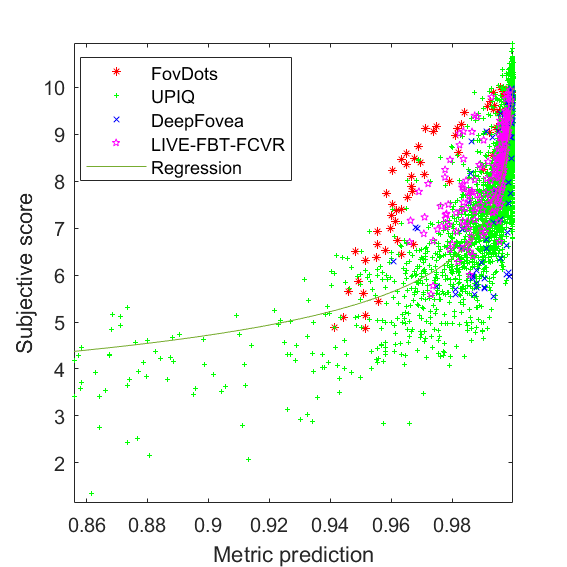JOD regression: Q = -0.399536 * z^1.24912 + 10, z=log2(1.001-M)

## LPIPS

RMSE = 1.174 PLCC = 0.7716 SROCC = 0.7554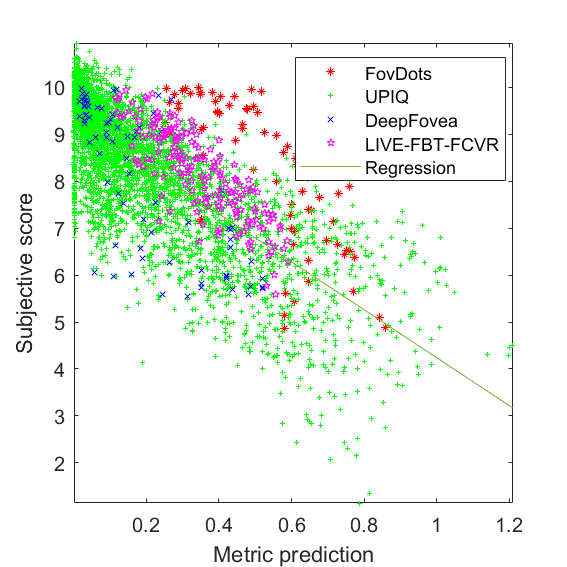JOD regression: Q = -5.10424 * M + 9.34396

## FWQI

RMSE = 1.21 PLCC = 0.6085 SROCC = 0.6191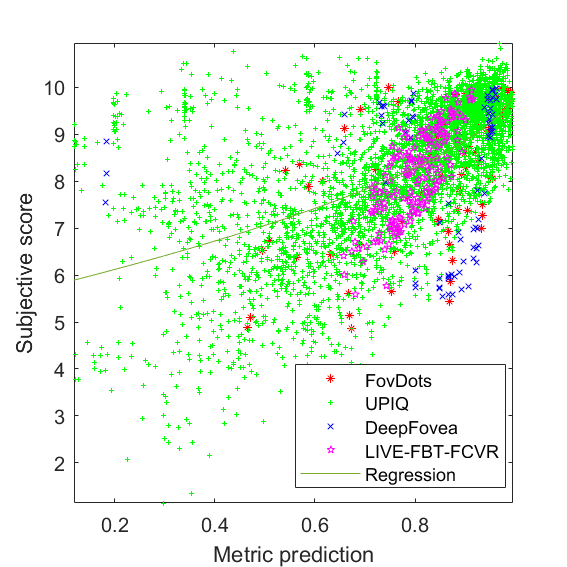JOD regression: Q = -4.42667*|1-M|^0.589672 + 10

## HDR-VDP-3 (3.0.6)

RMSE = 1.277 PLCC = 0.7432 SROCC = 0.7799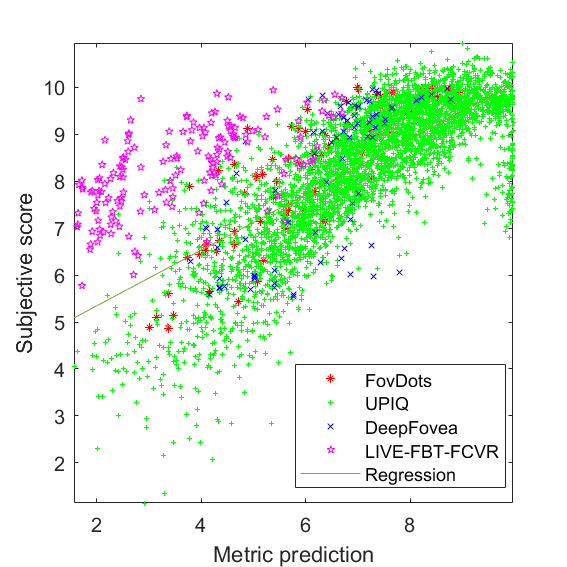JOD regression: Q = 0.593624 * M + 4.15607

## VMAF (v0.6.1 HDTV)

RMSE = 1.466 PLCC = 0.7841 SROCC = 0.7784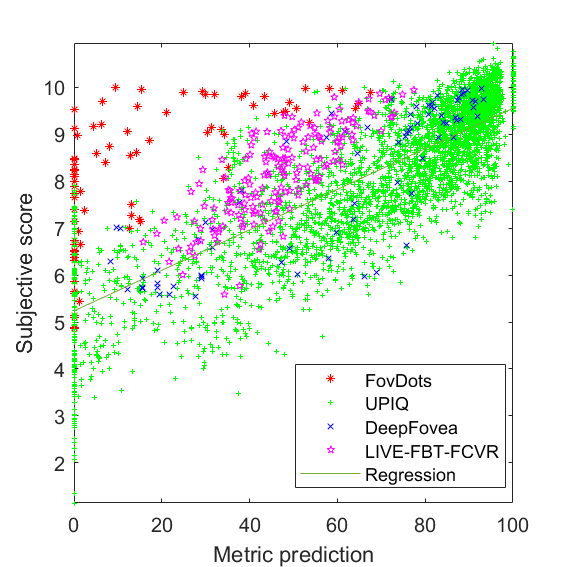JOD regression: Q = 0.0431542 * M + 5.23811